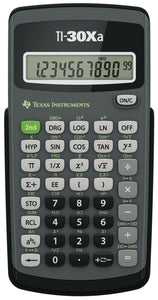# TI 30XA Solar School Edition Calculator

• \$14.99
Unit price per
Shipping calculated at checkout.

### Technical Information

Operations/Functionality:
• Fraction/decimal conversions
• Change improper fractions to mixed numbers
• Automatic simplification of fractions
• One constant
• Combinations and permutations
• Trigonometry
• Hyperbolics
• Logs and antilogs
• %, x²,x3, ¹/x, x?y, ?, x!
• Fixed decimal capability
• One-variable statistics
• AOS: (Algebraic Operating System)
• Three memory variables
• Scientific and engineering notation
• Polar/rectangular
• DMS/degrees
• Fraction/decimal
• Polar/rectangular
• DMS/degrees
• Fraction/decimal

### Display & Graphics

Number of Display Lines:   1
Number of Display Digits:   10
Display Screen Type:   LCD

### Controls/Indicators

Buttons & Keys:   Negation

### Battery Information

Power Source:   Battery